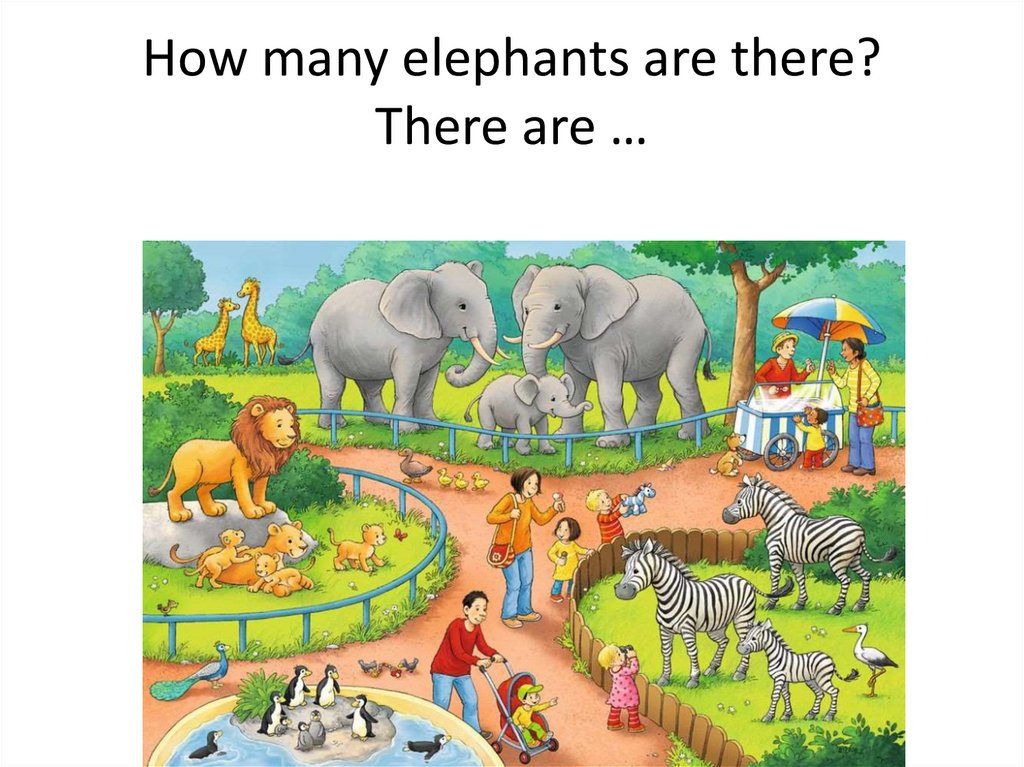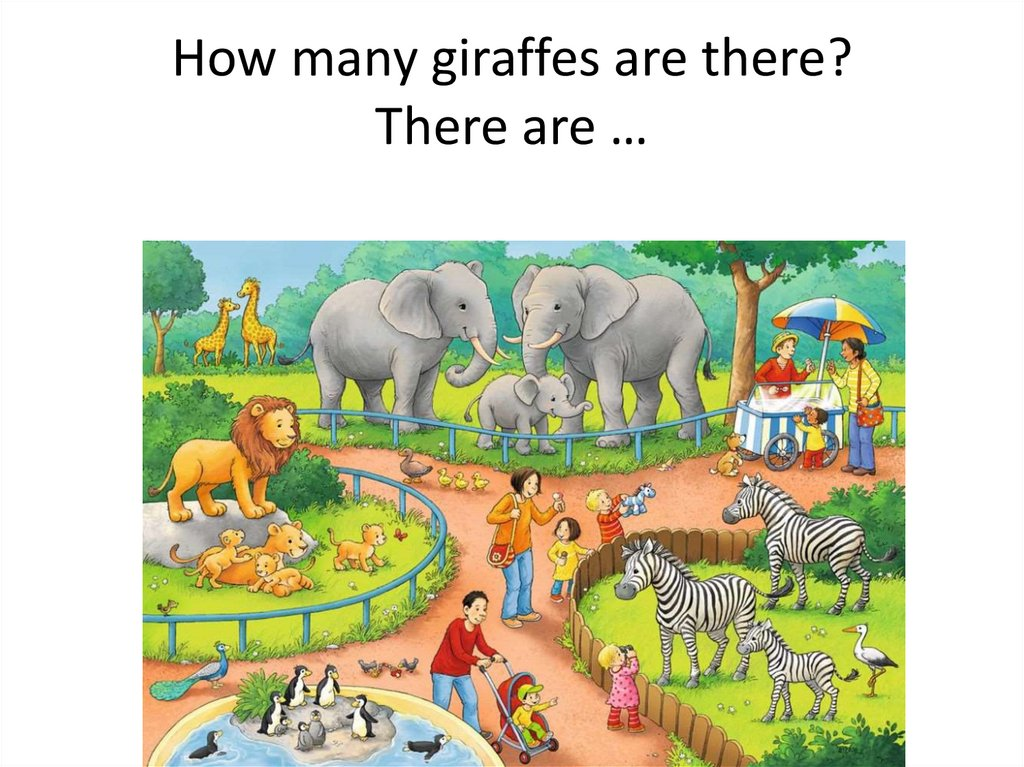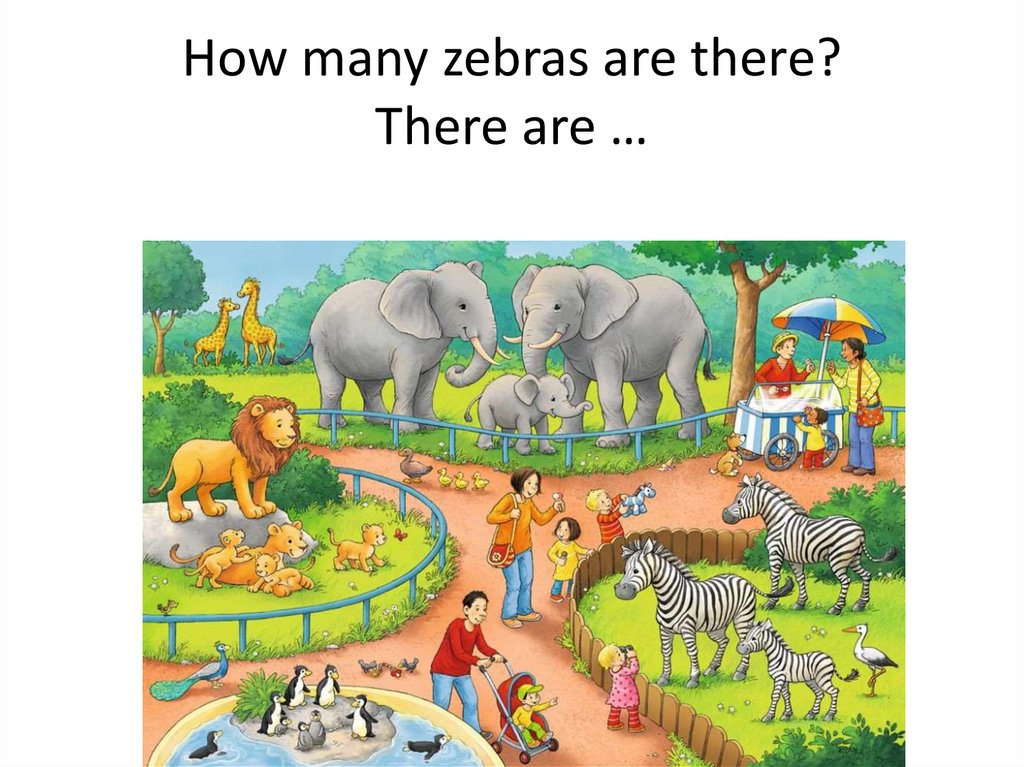# How many elephants are there?

## 1.

How many elephants are there?
There are …

## 2.

How many giraffes are there?
There are …

## 3.

How many zebras are there?
There are …

## 4.

How many lions are there?
There are …

## 5.

How many penguins are there?
There are …

## 6.

How many ducklings are there?
There are …

## 7.

How many giraffes are there?
There are …

## 8.

How many zebras are there?
There are …

## 9.

How many deer are there?
There are …

## 10.

How many parrots are there?
There are …

## 11.

How many elephants are there?
There are …

## 12.

How many penguins are there?
There are …

## 13.

How many polar bears are there?
There are …

## 14.

How many flamingos are there?
There are …

## 15.

How many monkeys are there?
There are …